# mathr / blog / #

## Haar wavelets

Quoth Wikipedia:

In mathematics, the Haar wavelet is a sequence of rescaled "square-shaped" functions which together form a wavelet family or basis. Wavelet analysis is similar to Fourier analysis in that it allows a target function over an interval to be represented in terms of an orthonormal basis. The Haar sequence is now recognised as the first known wavelet basis and extensively used as a teaching example.

The principle advantage of wavelet transform vs discrete Fourier transform is a different frequency/time localisation tradeoff: wavelets give better localisation in time for higher frequencies and better localisation in frequency for lower frequencies. This matches up better with human perception too.

The main advantage of 1D Haar wavelets in particular is that they are very easy to implement: given the source data block (power of two size), compute the sum and difference of each (non-overlapping) pair of consecutive samples. Put the sums in the first half of the array and the differences in the second half of the array, then repeat the process on the half containing the sums until there is only one sample. This process is reversible: no data is lost. I use a different normalization: dividing by two, so that the levels don't get higher in lower octaves. The DC bin then contains the mean instead of the sum. Source code in C:

// BLOCKSIZE must be a power of two
float array[BLOCKSIZE];

// compute Haar wavelet transform
// input/output in array using temporary array
for (int length = BLOCKSIZE >> 1; length > 0; length >>= 1)
{
for (int i = 0; i < length; ++i)
{
float a = array[2 * i + 0];
float b = array[2 * i + 1];
float s = (a + b) / 2;
float d = (a - b) / 2;
array[         i] = s;
array[length + i] = d;
}
for (int i = 0; i < BLOCKSIZE; ++i)
{
array[i] = array[i];
}
}

// compute inverse Haar wavelet transform
// input/output in array using temporary array
for (int length = 1; length <= BLOCKSIZE >> 1; length <<= 1)
{
for (int i = 0; i < BLOCKSIZE; ++i)
{
array[i] = array[i];
}
for (int i = 0; i < length; ++i)
{
float s = array[         i];
float d = array[length + i];
float a = s + d;
float b = s - d;
array[2 * i + 0] = a;
array[2 * i + 1] = b;
}
}

A classic FFT algorithm for audio is the "timbre stamp", applying the spectrum of one signal to another. A similar thing can be done with wavelets: compute the Haar wavelet transform of both signals, then compute the RMS energy per octave of the control signal. Multiply each octave of the carrier signal by the corresponding energy factor, then do compute the inverse Haar wavelet transform. As with FFT algorithms, windowed overlap-add resynthesis gives a smoother sound. Example audio snippet:

speech

The energy per octave representation is quite compact (for audio as humans can hear it it only makes sense to compute around 10 octaves), but even so it can give quite a lot of information about a signal. But most interesting signals are not stationary: the energy per octave changes over time. One simple statistical metric that can be used to quantify amount of change is the standard deviation (which is the square root of variance: variance is the expected value of the squared differences from the mean) of each octave bin.

Variance captures deviations from a mean value, but it doesn't capture much about how they vary. One idea I implemented was to compute another set of Haar wavelet transforms, this time capturing how the energy in each octave changes over time. Eventually I will subtract the mean from each energy vector before windowing, to avoid modulating the DC bin, but for now I just used a rectangular window. I then calculated the transform of each octave separately, then computed the RMS energy of each octave of "rhythm". This gives a 2D representation of energy vs octave (audio) vs octave (rhythm). Comparing various source audio files: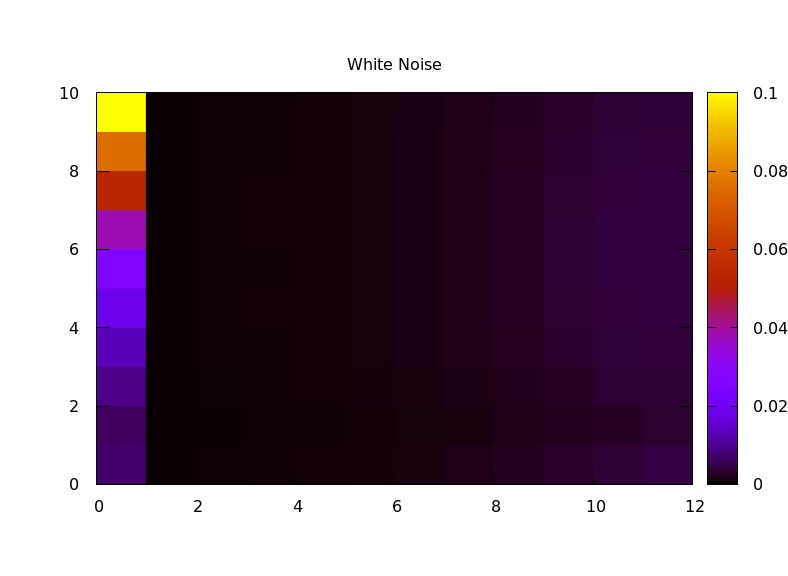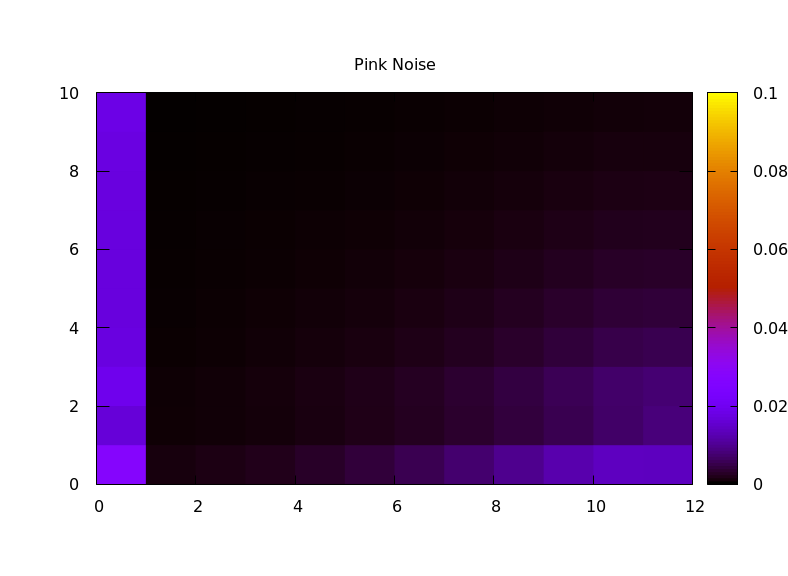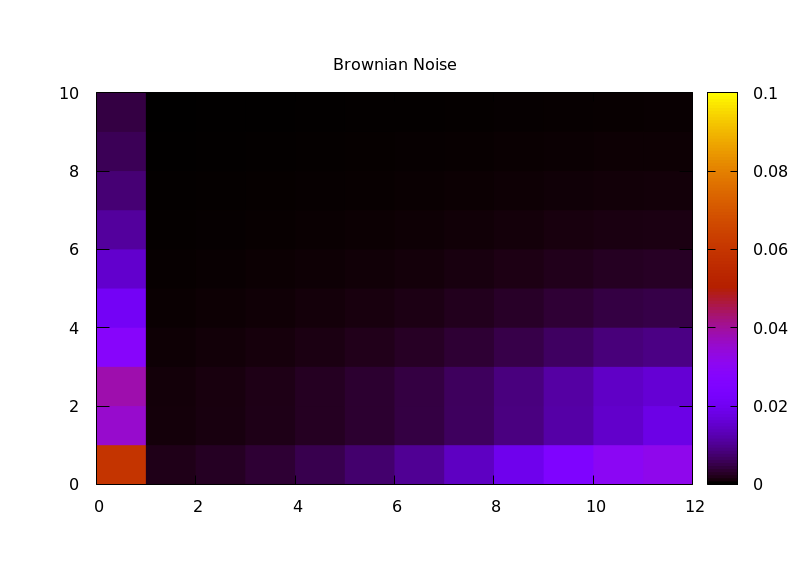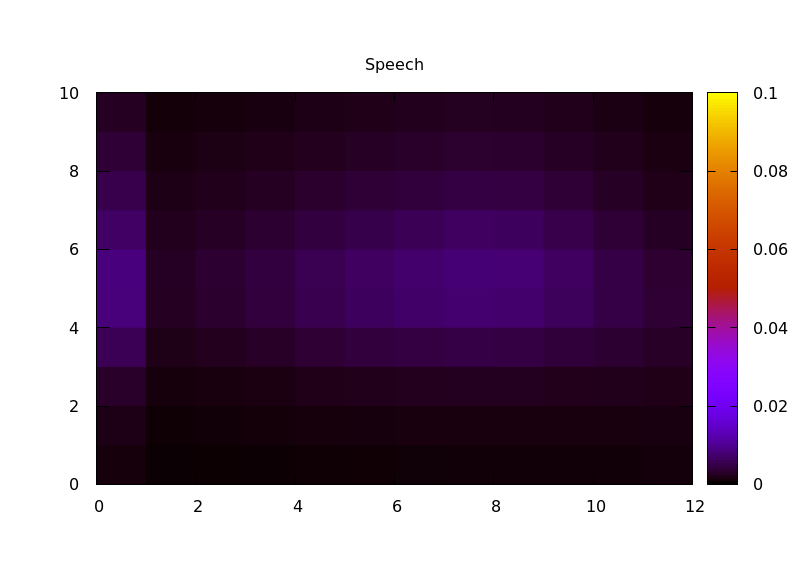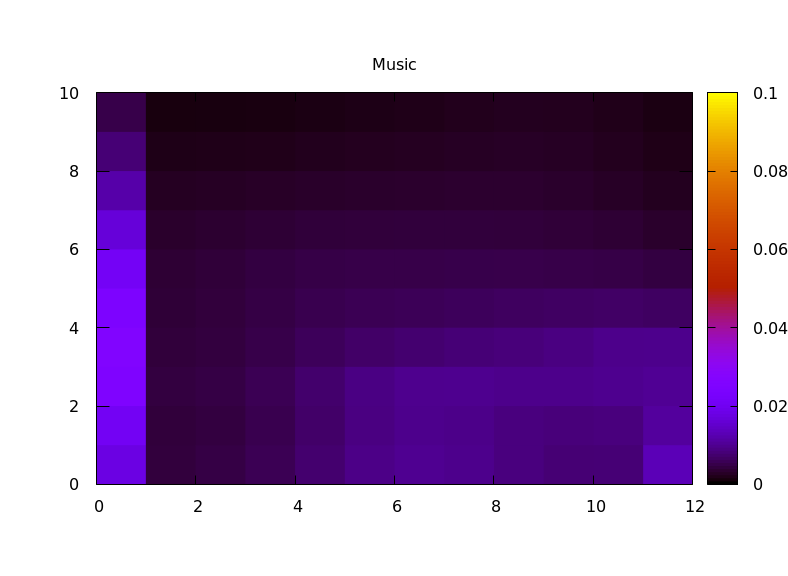The result seems like it might work reasonably well as an audio fingerprint. One next step in my research will be trying that out, to see if I can identify different audio files from a short snippet.

I tried reversing the process, going from the rhythm/octave fingerprint to audio, by stamping it on white noise. But it didn't work very well: the white noise had no energy in its rhythms (apart from DC), so amplifying zero still gives zero. I tried a feedback process but that amplified the DC-rhythm energy-per-octave spectrum over and over, so the result was very Alvin Lucier I am sitting in a room. A different approach that showed promise was normalizing the control rhythm/octave print first, and a variation involving normalizing the rhythm/octave print of the carrier is something I want to try next. Example audio snippets:

white

pink

brown

speech

music

One problem with the energy per octave (rhythm) representation is that it doesn't capture interactions between different octaves over time. One way of modelling sequential relationships is a Markov chain: it typically operates on discrete symbols at discrete time steps, and the probability of the next symbol generated depends on the recent history of generated symbols. Training a Markov chain is quite simple, but converting energy per octave vectors to symbols is not so easy. I ended up training a 2D self-organizing map to reduce the 11D vectors to a small 2D grid (8x8), and the index into the grid becomes the symbol for a first-order Markov chain. Then I generate symbols with the Markov chain and timbre stamp noise with the corresponding node vectors from the self-organizing map. For bonus points I could in future try Earth-mover's distance instead of regular Euclidean distance for the SOM metric. Example audio snippets:

white

pink

brown

speech

music

References for source audio (all 44100Hz stereo 16bit):

white
White noise generated with Audacity, 10mins
pink
Pink noise generated with Audacity, 10mins
brown
Brownian noise generated with Audacity, 10mins
speech
BBC Radio 4 - The Archers Omnibus 27/10/2019 76mins
music
CPI - Every Stone Held (2019) 95mins

A website for this project, including links to source code, is at mathr.co.uk/disco.